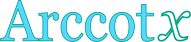## Inverse cotangent calculator

This tool evaluates the inverse cotangent of a number: arccot(x). The principal branch is evaluated, where the return values range between 0 and π.

 x = Result: arccot(x) = deg rad## Definitions

### General

The inverse cotangent function, in modern notation written as arccot(x), gives the angle θ, so that:

Due to the periodical nature of the cotangent function, there are many angles θ that can give the same cotangent value (i.e. θ+π, θ+3π, etc.). As a result, it is impossible to define a single inverse function, unless the range of the return values is restricted, so that a one-to-one relationship between θ and cotθ can be established. Therefore, multiple branches of the arccot function can be defined. Commonly, the desired range of θ values spans between 0 and π. The branch of arccot, in that case, is called the principal branch.

### Series

The arccot function can be defined in a Taylor series form, like this:

From the expanded form of the series, it can be seen that the higher terms become insignificant, for values of x close to zero, resulting in the following quite useful approximation:

### Properties

The derivative of the arccot function is:

The integral of the arccot function is given by:

The following properties are also valid for the arccot function: